• 安装&设置
• 数组和矩阵
• 输入输出
• 函数图像
• 逻辑和循环结构
• 未完待续

## 安装&设置

>> s = settings;s.matlab.desktop.DisplayScaleFactor
>> s.matlab.desktop.DisplayScaleFactor.PersonalValue = 1.5


## 数组和矩阵

Matlab 和 Python 有些类似，变量并不需要声明便可以直接赋值。

>> a = [1 2 3]


>> a(2)


ans =

2


>> a(2) = 10


>> a = [1:10:101]


>> a = [1:10]


>> A = [1 0 0; 0 5 0; 1 0 0.5]


Matlab 还可以轻松的将两个矩阵拼接到一块：

>> [A, A]
>> [A; A]


$\det A$det(A)

$A^{-1}$inv(A)A^-1

$A^{T}$A'

$A^k$A^k

$AB$A*B

$A+B$A+B

$A-B$A+B

Matlab 还支持复数运算，其中虚数单位用 ij 表示：

>> i^2
>> j^2


>> format short
>> 1.1

ans =

1.1000

>> format long
>> 1.1

ans =

1.100000000000000



## 输入输出

>> str = "The number is " + 10 + "."


>> A = ["a","bb","ccc"; "dddd","eeeeee","fffffff"]


>> strlength(A)

ans =

1     2     3
4     6     7


>> a = 'abc';
>> a(1)

ans =

'a'


>> x = input("Please input a number: ");


>> x = input("Please input a string: ",'s');


>> disp("Hello, world!");


## 函数图像

x = linspace(-10, 10, 51); % 在区间[-10,10]中均匀选择51个点构成数组，包括-10和10
y = x.^2;
plot(x,y); % 画图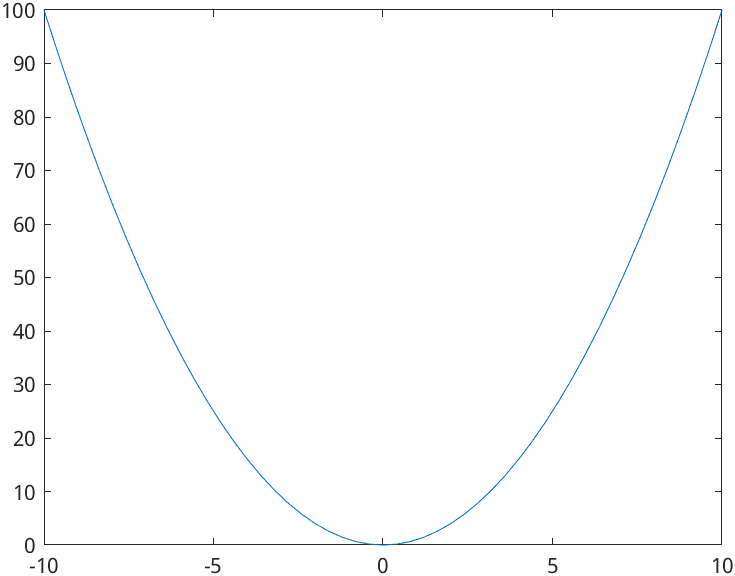x = linspace(-10, 10, 51);
y = x.^2;
plot(x, y);
xlabel("x");
ylabel("x^2");
title("Plot of the parabola");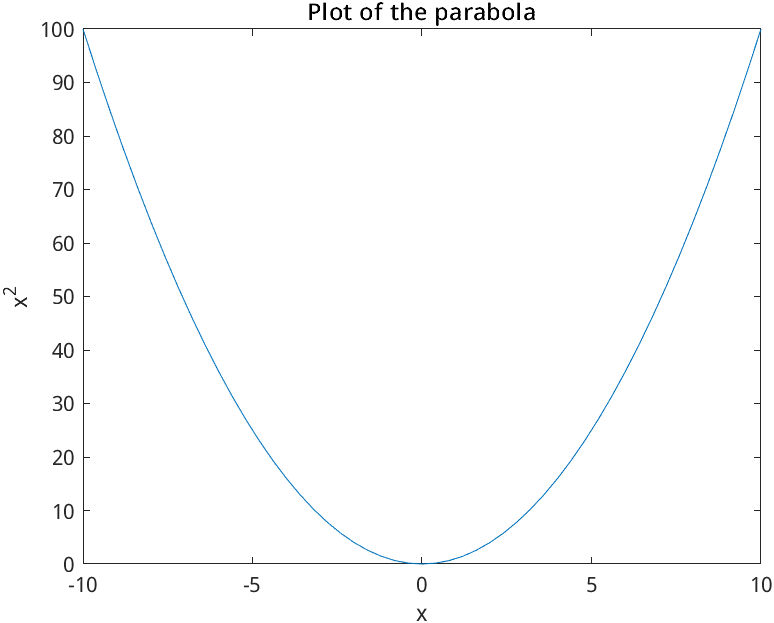x = linspace(-10, 10, 51);
y = x.^2;
plot(x, y, "r--");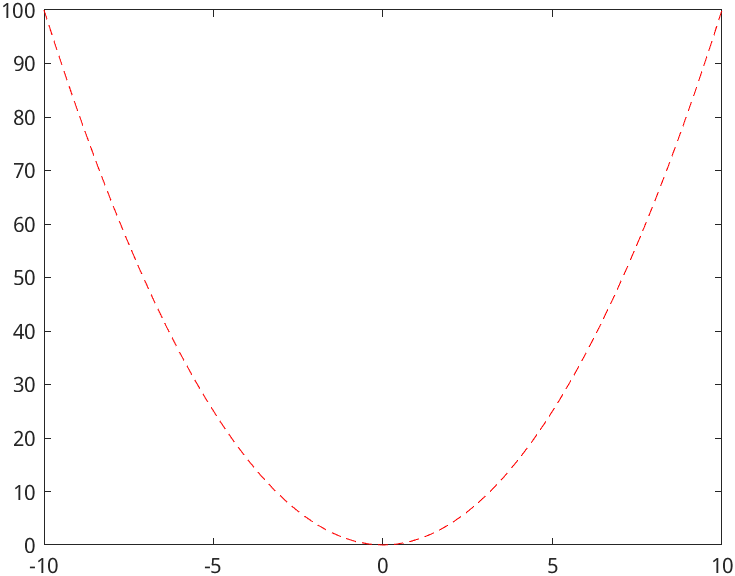x = linspace(-2, 2, 50);
y = x.^2;
plot(x, y, "r--");
hold on; % 在同一个图像中画图需要使用hold on
plot(y, x, "b");
legend("y=x^2","y^2=x"); % 标记两条曲线的名称
hold off; % 结束画图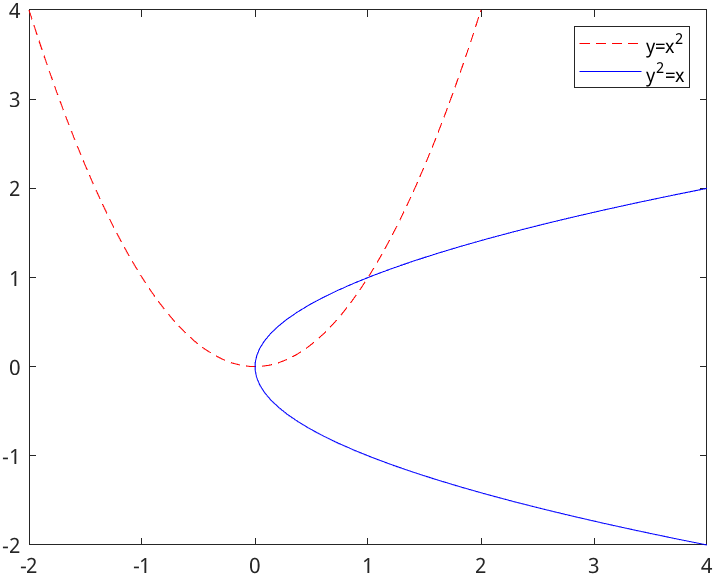t = tiledlayout(1,2); % 布局，1行2列
title(t,"Plots"); % 最上方的大标题

nexttile;
x = linspace(-2, 2, 50);
y = x.^2;
plot(x, y);
title("y=x^2");

nexttile;
plot(y, x);
title("y^2=x");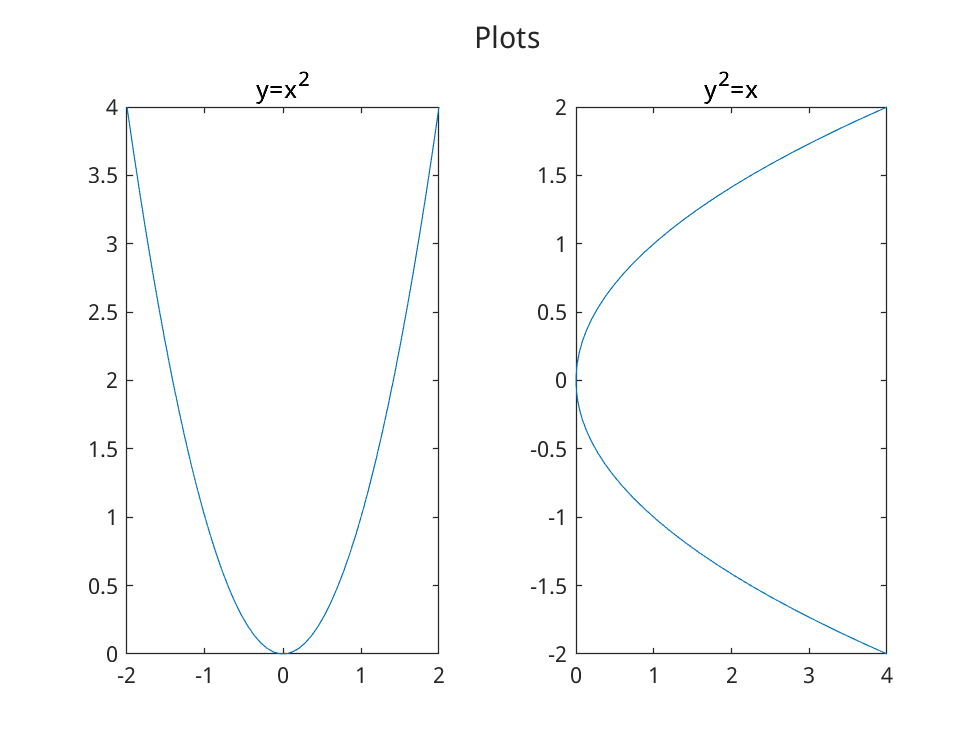x = linspace(-5,5,20);
y = x'; % y 座标的矩阵（只有一列的矩阵）为 x 座标矩阵的转置
z = x.^2/25-y.^2/16;
surf(x,y,z); % 三维绘图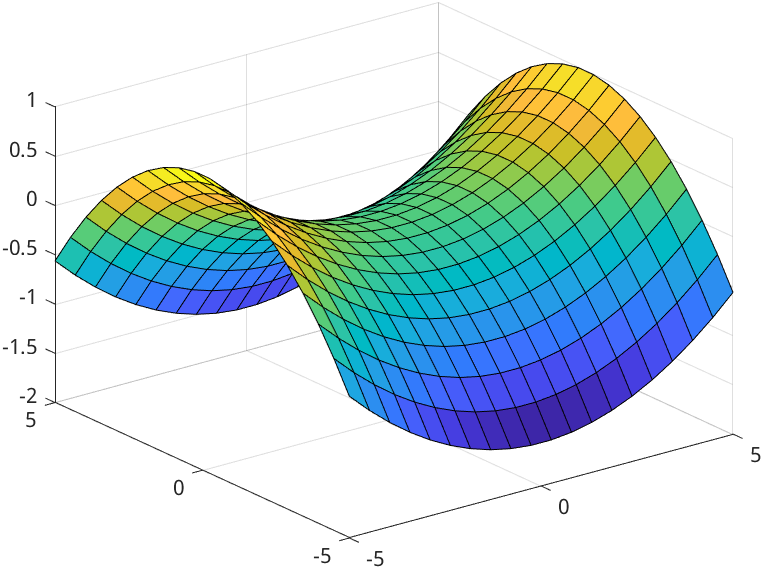## 逻辑和循环结构

N = 100;
f(1) = 1;
f(2) = 1;

for n = 3:N % 按顺序将 n 赋值为数组 3:N 中的每一个元素并执行后面的语句
f(n) = f(n-1) + f(n-2);
end
f(1:10)


num = randi(100); % 生成一个 [1,100] 内的整数随机数
if num < 34
sz = 'low';
elseif num < 67
sz = 'medium';
else
sz = 'high';
end
disp(num + " is " + sz);


## 评论

1.小浅
1年前
2022-1-07 18:42:30

第一%

2.迷妹
12月前
2022-2-16 0:16:11

嘿嘿嘿

## 发送评论编辑评论

|´・ω・)ノ
ヾ(≧∇≦*)ゝ
(☆ω☆)
（╯‵□′）╯︵┴─┴
￣﹃￣
(/ω＼)
∠( ᐛ 」∠)＿
(๑•̀ㅁ•́ฅ)
→_→
୧(๑•̀⌄•́๑)૭
٩(ˊᗜˋ*)و
(ノ°ο°)ノ
(´இ皿இ｀)
⌇●﹏●⌇
(ฅ´ω`ฅ)
(╯°A°)╯︵○○○
φ(￣∇￣o)
ヾ(´･ ･｀｡)ノ"
( ง ᵒ̌皿ᵒ̌)ง⁼³₌₃
(ó﹏ò｡)
Σ(っ °Д °;)っ
( ,,´･ω･)ﾉ"(´っω･｀｡)
╮(╯▽╰)╭
o(*////▽////*)q
＞﹏＜
( ๑´•ω•) "(ㆆᴗㆆ)

Emoji